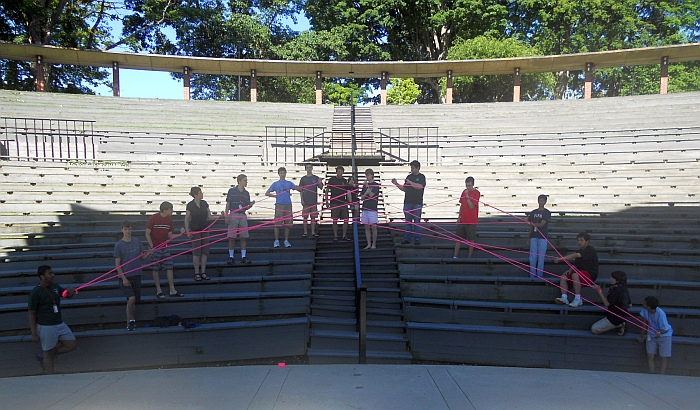By George Hart for the Museum of MathematicsA complete graph is what mathematicians call a collection of items in which every pair is connected. If the items are spaced evenly around a circle and the connections are shown as straight lines, the lines form an attractive pattern of concentric circles.This is a complete graph with eleven vertices. We made it at Mathcamp 2010 using plastic surveyor’s tape. There is a simple algorithm for constructing it in which people stand in a circle, and pass the roll from one to the next while counting aloud, wrapping it around their left wrist at the proper intervals.A similar algorithm, executed by people standing in two straight lines, gives this large parabola. The construction steps for both of these figures can be seen in the additional photos here.

More:
See all of George’s Math Monday columns

## 12 thoughts on “Math Monday: Human-scale string constructions”

1.http://david.rysdam.org/blog/ says:

“Above is the standard axes orientation. Can you prove these lines are tangent to a parabola?”

No, because they aren’t, I don’t think. The figure shown is a quarter circle. If you linearly distorted one axis, you’d have a section of an ellipse (because a circle is a special, undistorted ellipse). If NON-linearly distorted one axis in the right way, then you’d have (part of) a parabola.

I know this because just last week my officemates and I figured out the formula for the non-linear distortion.

2.George Hart says:

The “envelope of the lines” is a parabola. I am assuming people stand at integer points on the axes and there is a segment from (x,0) to (0,y), where x+y=c. (c is a constant, roughly half the number of people available.) For example, with c=10, there could be a segment from (5,0) to (0,5), and one from (6,0) to (0,4), and generally from (n,0) to (0, 10-n). For very large or small n, these tangent lines approach a 45 degree slope, so they become parallel, which is characteristic of a parabola.

3.http://david.rysdam.org/blog/ says:

(n,0) to (0, 10-n). For very large or small n, these tangent lines approach a 45 degree slope, so they become parallel, which is characteristic of a parabola.

For large and small n, the slopes would approach 0 and 90 degrees, respectively. I.e. with c = 100 and n = 99 (large), a line from (99,0) to (0,1) would be almost horizontal while for small n = 1, a line from (1,0) to (0,99) would be almost vertical.

But even if you were right, I don’t see how that makes this figure a parabola. If you assume equal spacing on the x and y axes, then by symmetry it can’t be a parabola. Swapping the axes does nothing to the envelope, while swapping the axes of a parabola *does* do something.

4.http://david.rysdam.org/blog/ says:

That first part is me quoting you. My remarks begin with “For large and small n, the slopes would approach 0 and 90…”

Also, I always check the “Email me if someone responds” and never get emails. What’s up with that?

5.George Hart says:

You are thinking of the familiar y=x^2 parabola, which has its line of mirror symmetry vertical, because the mirror line is the Y axis. But if a parabola is moved, scaled, or rotated it is still a parabola. For the integer coordinates I used in explaining the string figure parabola above, the line of mirror symmetry is the line x=y of slope 1. The string parabola is then symmetric in X and Y. It is rotated 45 degrees clockwise from the y=x^2 parabola. Then, to understand how the slope of the tangent is changing as n gets very large or small, you must pick any constant c, keep it fixed and vary n.

6.http://david.rysdam.org/blog/ says:

I’m going to have to look at this closer to try to align my understanding with yours.